# 类的静态成员

## 一.知识要点——静态数据成员

1.静态成员是解决同一个类的不同对象之间数据和函数共享问题的。
2.静态成员在每一个类中只有一个副本，由该类的所有对象共同维护使用，从而实现了同一个类的不同对象之间的数据共享。
3.使用static关键字来声明为静态成员
4.类的静态成员需要在类的定义之外在加以定义
5.由于静态数据成员不属于任何一个对象，因此可以通过类名对它进行访问，一般的用法是“类名：：标识符”

## 二.代码分析

（教材155页）

#include <iostream>
using namespace std;

class Point {
public:
Point(int X=0,int Y=0)
{x=X;
y=Y;
count++;
}//构造函数

Point(Point &p)
{x=p.x;
y=p.y;
count++;
}//复制构造函数
~Point(){count--;}//析构函数

int getx(){return x;}
int gety(){return y;}
void showCount(){
cout<<"count="<<count<<endl;}
private:
int x,y;
static int count;//静态数据成员的声明
};

int Point::count=0;//静态数据成员定义和初始化

int main()
{
Point a(4,5);
cout<<"Point a="<<a.getx()<<","<<a.gety()<<endl;
a.showCount();

Point b(a);
cout<<"Point b="<<b.getx()<<","<<b.gety()<<endl;
b.showCount();

return 0;
}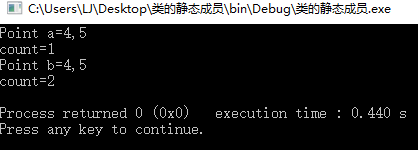1.修改代码：这里我们把类的静态成员count在类外的定义注释掉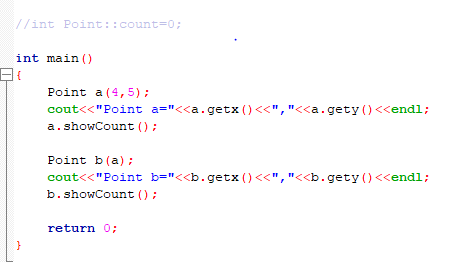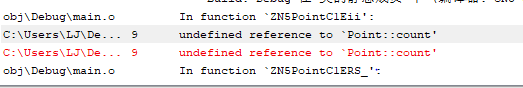说明：静态数据成员需要我们在类外以专门的方式为他们分配空间，也证明了静态数据成员它是不属于任何一个对象的。它不会像非静态成员那样，在给对象分配空间的同时也给非静态成员分配了空间。

2.修改代码：这里我们把静态数据成员count变成非静态数据成员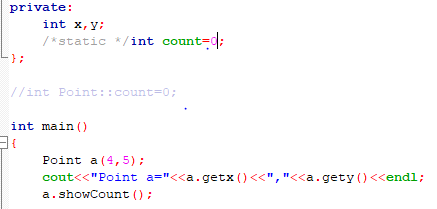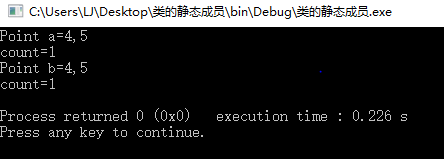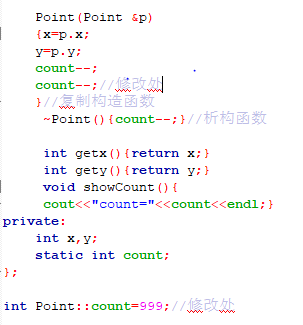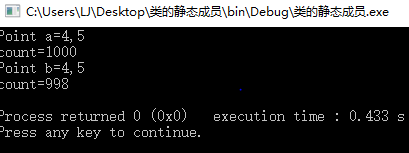分析上面两次的修改，在count是非静态数据成员的时候，两个对象a b分别访问的是不同的count,他们都在最初的count上进行了加一。而如果count是静态数据成员，a b两个对象访问的将是同一个静态成员，b对象中count的值的变化也是建立在a变化以后的，而并非是在定义的999这个值上进行变化的。

## 一.知识要点——静态函数成员

1.静态成员函数就是使用static关键字声明的函数成员，同静态数据成员一样，静态成员函数也属于整个类，由同一个类的所有对象共同拥有，为这些对象所共享。
2.静态成员函数可以直接访问该类的静态数据和函数成员，而访问非静态成员必须通过对象名

## 二.代码分析

（教材157页158页）

#include <iostream>
using namespace std;

class Point {
public:
Point(int X=0,int Y=0)
{x=X;
y=Y;
count++;
}//构造函数

Point(Point &p)
{x=p.x;
y=p.y;
count++;
}//复制构造函数
~Point(){count--;}//析构函数

int getx(){return x;}
int gety(){return y;}

static void showCount()
{cout<<"count="<<count<<endl;}//静态函数成员

private:
int x,y;
static int count;
};

int Point::count=0;

int main()
{
Point a(4,5);
cout<<"Point a="<<a.getx()<<","<<a.gety()<<endl;
Point::showCount();//调用静态函数成员

Point b(a);
cout<<"Point b="<<b.getx()<<","<<b.gety()<<endl;
Point::showCount();//调用静态函数成员

return 0;
}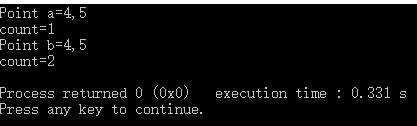1.修改代码：我们把静态函数成员改成非静态函数成员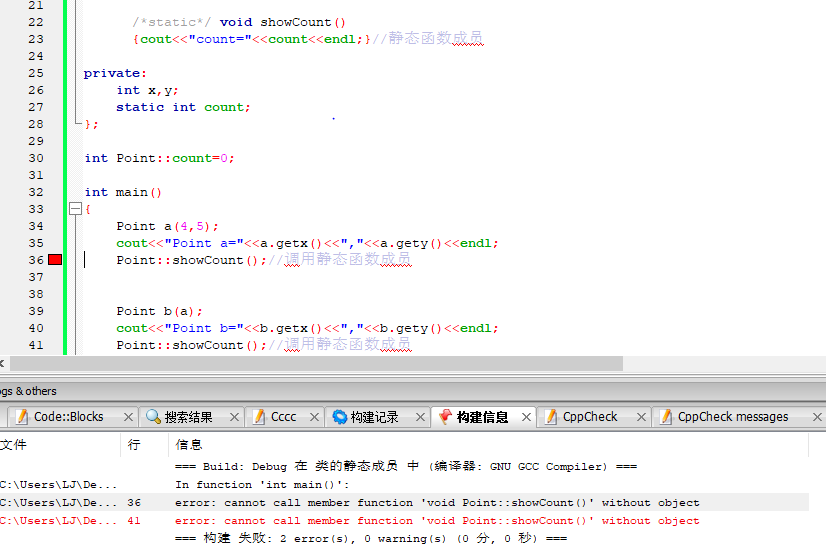编译时候发生的错误说明对于普通函数成员的调用必须咬通过对象名，而对于静态成员函数的调用我们可以直接通过类名来进行调用

posted on 2019-09-29 19:36  咬一口云朵软绵绵  阅读(219)  评论(0编辑  收藏  举报